### Pseudo-Euclidean embedding

This page belongs to the User Guide of the DisTools Matlab package. It describes some of its commands. Links to other pages are listed above. More information can be found in the pages of the PRTools User Guide. Links are given at the bottom of this page.

By embedding a dissimilarity matrix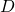a vector space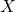with a given norm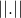is constructed such that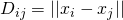. Obviously, by definition, the dissimilarity matrix has to be Euclidean for a perfect embedding into an Euclidean vector space. A more general space is the pseudo-Euclidean space in which any symmetric dissimilarity matrix with a zero diagonal can be perfectly embedded. Even off-diagonal zeros and negative numbers are allowed.

The Pseudo-Euclidean space (PE-space)  consists of two orthogonal Euclidean subspaces, called the positive space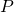and the negative space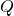. By embedding, vectors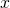are constructed in which the first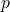components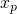refer toand the followingcomponentsto,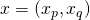. Usually, for non-degenerate (full rank) dissimilarity matrices holds that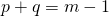ifdescribes the dissimilarities betweenobjects. The pseudo-Euclidean norm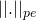is now defined as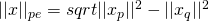.

The PE-space can be interpreted as a Euclidean spaceoffering an approximate solution for the embedding and a spacein which all corrections are collected. Distances (or the length of vectors) are now computed (in the squared sense) by subtracting the corrections from the Euclidean norm (distances). Consequently, squared distances may be zero or even negative, resulting in a complex norm.

The main DisTools routine for PE- embedding is called `pe_em`. It contains various options for returning reduced spaces of a lower dimensionality. The mapping produced by the routine may be used to project the original training data on the pe_space, or, alternatively, any other dissimilarity matrix based on the same representation set. It should be realized this can be very inaccurate for non-Euclidean dissimilarities and high-dimensional spaces, resulting in imaginary distances in the PE-space in cases for which the given distances are all positive.

In addition to `pe_em` there are various other quantities describing the non-Euclidean characteristics of a dissimilarity matrix.

• The signature is the duplet (p,q) describing the dimensionalities of the positive and the negative space.
• The spectrum is the set of ranked eigenvalues of a Gramm matrix computed from the dissimilarity matrix. Some eigenvalues are negative in case of non-Euclidean dissimilarities. The eigenvectors related to the positive eigenvectors correspond with the axes of the positive space and the negative ones with the negative space.
• The Non-Euclidean Fraction, NEF, considers the absolute values in the spectrum. The sum over the eigenvalues found for the negative space divided by the total sum over all eigenvalues constructs the NEF.
• The Non-Metricity Fraction, NMF, is the fraction of triplets in the dissimilarity matrix (corresponding to the dissimilarities between three objects) that violates the the triangle inequality: the largest of the three is larger than the sum of the two other ones.

In the below table a number of DisTools commands and small examples is listed. They may be applied to a simple non-Euclidean dissimilarity matrix, e.g. as generated in the first rows:

 ```A = gendatb; D = A*proxm(A,'m',1);``` Generate a 2-dimensional dataset, 2 classes, 100 objects. Compute the 100 x 100 dismat based on Minkowsky-1 metric. This dissimilarity is metric but non-Euclidean. ```A = gendatb; D = A*proxm(A,'m',1); D = D.^5;``` Generate a 2-dimensional dataset, 2 classes, 100 objects. Compute the 100 x 100 dismat based on Minkowsky-1 metric. Take the 5th power, creating a dissimilarity which is non-metric. ```W = D*pe_em; X = D*W;``` Compute PE mapping Apply to dissimilarity matrix `[DT,DS] = genddat(D,0.5);` ` W = DT*pe_em;` ` XT = DT*W;` ` XS = DS*W;` Split given dataset in trainset and testset Compute PE mapping using the trainset Map the trainset Map the testset `W = D*pe_em;` ` X = D*W` `W*signature` ` X*``signature` ` XA= X*euspace;` ` XP= X*euspace([],'pos');` ``` XN= X*euspace([],'neg'); X*nef XA*nef W*nef``` Compute PE mapping Apply mapping to dissimilarity matrix Retrieve the signature of `W` Retrieve the signature from the PE space Convert x to associated space Convert x to positive space Convert x to negative space Compute negative eigenfraction from `X` Compute negative eigenfraction from XA Compute negative eigenfraction from `W` `D*nmf` ` D.^3*nmf` Compute non-metric fraction of `D` Compute non-metric fraction of `D.^3` ```W = D*pe_em; S = getspectrum(W); plotspectrum```(W) Compute a PE mapping Get its spectrum PLot its spectrum

In the next table a number of routines and classifiers are listed that make use of the specific norm that is defined for a PE space. This norm follows from the signature and is stored in a dataset x that is projected in a PE space by mappings that are computed by `pe_em: X = D*(D*pe_em); or X = D*pe_em(D);`

 `D = pe_distm(X,Y)` Square PE dismat between two datasets `Z = pe_mtimes(X,Y)` Matrix multiplication (inner product) in PE space `K = X*pe_kernelm` Compute linear kernel in PE space `W = X*pe_nmc` Nearest mean classifier in PE space `W = X*pe_knnc` KNN classifier in PE space `W = X*pe_parzenc` Parzen classifier in PE space `W = X*pe_libsvc` SVM in PE space

Here is a small example that splits a dataset in a trainset and a testset and computes the performances for three classifiers

`A = gendatb;`
`D = A*proxm(A,'m',1);`
`W = D*pe_em;`
`X = D*W;`
`[XT,XS] = gendat(X,0.5);`
`W = XT*{pe_nmc,pe_knnc,pe_parzenc,pe_libsvc};`
`testc(XS,W)`